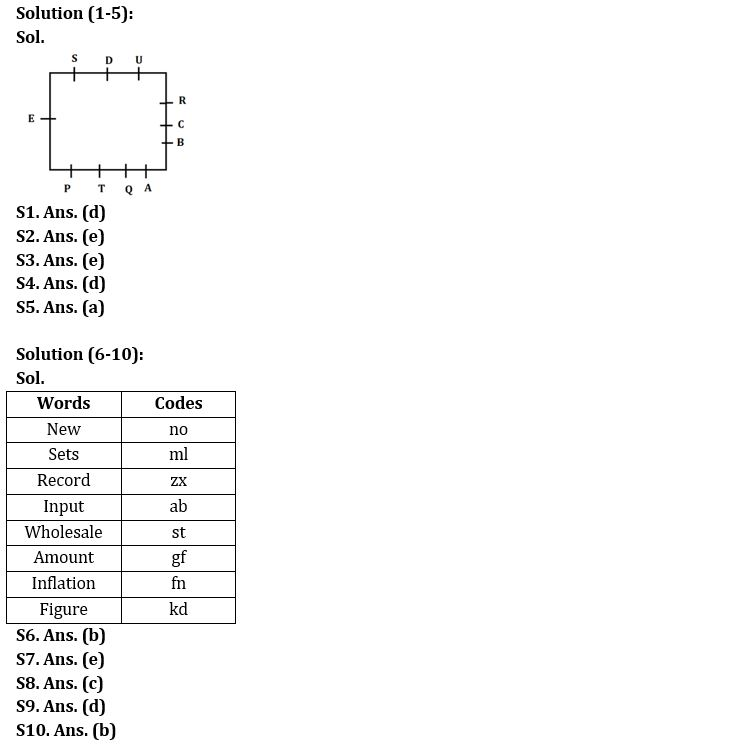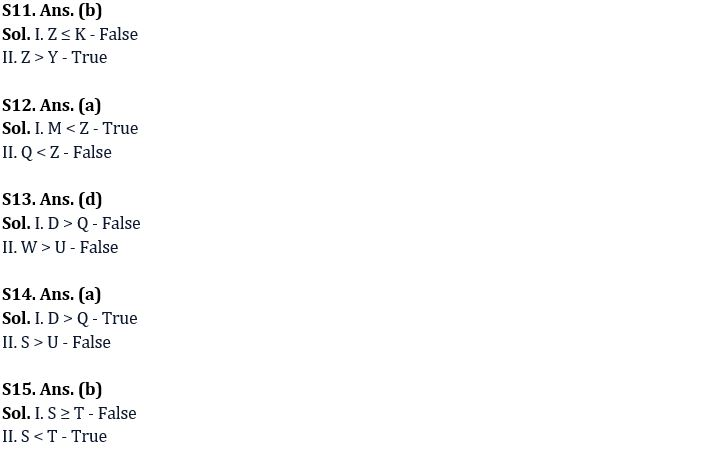Latest Banking jobs   »

# Reasoning Ability Quiz For IBPS Clerk Prelims 2022- 8th August

Directions (1-5): Answer the questions based on the information given below.
Eleven persons A, B, C, D, E, P, Q, R, S, T, and U sit around a square table facing the center of the table such that four persons on one side, three on each two sides and one person on the remaining side of the table. A. P, Q, and T sit on the side on which four persons are sitting.
Note: The sides on which three persons are sitting are adjacent to each other. The side in which only one person is sitting is immediate left of the side along which four persons are sitting.
R sits 3d to the right of A. E sits 4th to the right of R. S sits immediate left of E. T sits 3rd to the right of S. P does not sit adjacent to A. B sits 4th to the left of D. U does not sit adjacent to B.

Q1. What is the position of A with respect to E?
(a) 2nd to the left
(b) 2nd to the right
(c) 3rd to the left
(d) 4th to the right
(e) Can’t be determined

Q2. Who sits 2nd to the left of T?
(a) R
(b) Q
(c) S
(d) P
(e) E

Q3. How many persons sit between D and Q when counted from the right of D?
(a) Three
(b) One
(c) Two
(d) None
(e) Four

Q4. What is the position of B with respect to U?
(a) Immediate left
(b) 2nd to the left
(c) Fourth to the left
(d) 3rd to the left
(e) None of these

Q5. Which of the following statement is/are correct?
(a) U sits immediate right of R
(b) R sits immediate right of B
(c) E sits 2nd to the right of B
(d) A sits immediate right of T
(e) All are correct

Directions (6-10): Answer the questions based on the information given below.
In a certain code language:
‘New record sets input’ is written as ‘no ml zx ab’,
‘Record sets wholesale amount’ is written as ‘ml zx st gf’
‘Figure wholesale amount inflation’ is written as ‘kd fn gf st’
‘Record input figure amount and ‘zx ab gf kd’

Q6. What is the code for ‘figure’ in the coded language?
(a) ab
(b) kd
(c) zx
(d) gf
(e) Either zx or gf

Q7. If ‘market wholesale amount’ is coded as ‘st gf op’ then what may be the code for ‘market record’ in the given coded language?
(a) zx gf
(b) op ab
(c) zx fn
(d) op st
(e) op zx

Q8. What is the code for ‘New’ in the given coded language?
(a) zx
(b) ab
(c) no
(d) ml
(e) None of these

Q9. What is the code for ‘Record sets’ in the given coded language?
(a) zx gf
(b) ml st
(c) ab zx
(d) ml zx
(e) None of these

Q10. Which of the following word is coded as ‘fn’?
(a) Input
(b) Inflation
(c) Figure
(d) Record
(e) None of these

Directions (11-15): In the following question assuming the given statements to be true, find which of the conclusion among given conclusions is /are definitely true and then give your answers accordingly.

Q11. Statements: K ≥ C ≥ X < F = Z; X > D > Y
Conclusions: I. Z ≤ K
II. Z > Y
(a) Only I is true
(b) Only II is true
(c) Either I or II is true
(d) Neither I nor II is true
(e) Both I and II are true

Q12. Statements: P = M < O ≤ Q ≤ T = Z > I
Conclusions: I. M < Z
II. Q < Z
(a) Only I is true
(b) Only II is true
(c) Either I or II is true
(d) Neither I nor II is true
(e) Both I and II are true

Q13. Statements: D > S = I; I < Q < U; S < W = T
Conclusions: I. D > Q
II. W > U
(a) Only I is true
(b) Only II is true
(c) Either I or II is true
(d) Neither I nor II is true
(e) Both I and II are true

Q14. Statement: M > Q = U < D ≤ Z > S
Conclusion: I. D > Q
II. S > U
(a) Only I is true
(b) Only II is true
(c) Either I or II is true
(d) Neither I nor II is true
(e) Both I and II are true

Q15. Statement: D > S = Q < O ≤ T > R
Conclusions: I. S ≥ T
II. S < T
(a) Only I is true
(b) Only II is true
(c) Either I or II is true
(d) Neither I nor II is true
(e) Both I and II are true

Solutions#### Congratulations!Download Hindu Review of October 2021: Free PDF